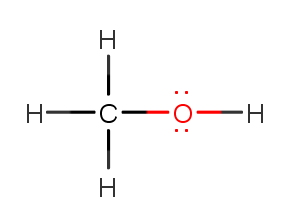# Problem: Determine the electron geometry, molecular geometry and polarity around the O of CH3OH .a) eg=tetrahedral, mg=bent, polarb) eg=linear, mg=linear, polarc) eg=trigonal bipyramidal, mg=trigonal planar, nonpolard) eg=tetrahedral, mg=linear, nonpolare) eg=octahedral, mg=square planar, nonpolar

###### FREE Expert Solution

Step 1: Carbon (EN = 2.5) is less electronegative than oxygen (EN = 3.5) so C is the central atom.

Step 2: The total number of valence electrons:

Group             Valence Electrons

C          4A                   1 × 4 e4 e

H         1A                   4 × 1 e4 e

O         6A                   1 × 6 e6 e

Total:  14 valence e

Step 3: O prefers 2 bonds. The complete Lewis structure of CH3OH is:100% (319 ratings)###### Problem Details

Determine the electron geometry, molecular geometry and polarity around the O of CH3OH .

a) eg=tetrahedral, mg=bent, polar

b) eg=linear, mg=linear, polar

c) eg=trigonal bipyramidal, mg=trigonal planar, nonpolar

d) eg=tetrahedral, mg=linear, nonpolar

e) eg=octahedral, mg=square planar, nonpolar

Frequently Asked Questions

What scientific concept do you need to know in order to solve this problem?

Our tutors have indicated that to solve this problem you will need to apply the Molecular Polarity concept. You can view video lessons to learn Molecular Polarity. Or if you need more Molecular Polarity practice, you can also practice Molecular Polarity practice problems.